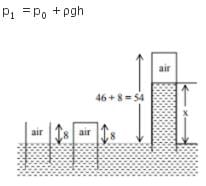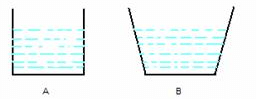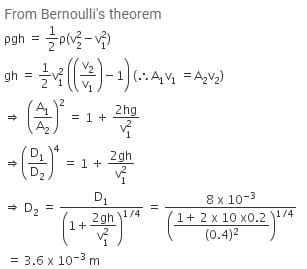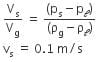NEET  >  Test: Pressure & Its Applications

# Test: Pressure & Its Applications

Test Description

## 10 Questions MCQ Test Physics Class 11 | Test: Pressure & Its Applications

Test: Pressure & Its Applications for NEET 2023 is part of Physics Class 11 preparation. The Test: Pressure & Its Applications questions and answers have been prepared according to the NEET exam syllabus.The Test: Pressure & Its Applications MCQs are made for NEET 2023 Exam. Find important definitions, questions, notes, meanings, examples, exercises, MCQs and online tests for Test: Pressure & Its Applications below.
Solutions of Test: Pressure & Its Applications questions in English are available as part of our Physics Class 11 for NEET & Test: Pressure & Its Applications solutions in Hindi for Physics Class 11 course. Download more important topics, notes, lectures and mock test series for NEET Exam by signing up for free. Attempt Test: Pressure & Its Applications | 10 questions in 10 minutes | Mock test for NEET preparation | Free important questions MCQ to study Physics Class 11 for NEET Exam | Download free PDF with solutions
 1 Crore+ students have signed up on EduRev. Have you?
Test: Pressure & Its Applications - Question 1

### The pressure of 1 atm = ______ Pa

Detailed Solution for Test: Pressure & Its Applications - Question 1

Pressure of 1 atm = 1.013 x 105 Pa

Test: Pressure & Its Applications - Question 2

### In a car lift, compressed air exerts a force F1​ on a small piston having a radius of 5cm. This pressure is transmitted to the second piston of a radius of 15cm. If the mass of the car to be lifted is 1350 kg. What is F1​?

Detailed Solution for Test: Pressure & Its Applications - Question 2

From Pascal’s law: P​= P2

⇒ F1/A​​= ​F2/A2​​
⇒ F1/πr1​2​ ​= F2/πr2​​
⇒ F1 = (F* r1​2 ) / r22  = 1350 * 9.8 * (5 * 10-2)2 / (15 * 10-2)2 = 1470 N ​= 1.47 * 10N

Test: Pressure & Its Applications - Question 3

### Which of the following is not an application of Pascal’s Law?

Detailed Solution for Test: Pressure & Its Applications - Question 3

Pascal's law states that the magnitude of pressure within the fluid is equal in all parts.

Option A: Brahma's press is a Hydraulic Press that works on the principle of Pascal's law.

Option B: Submarines don't work on the principle of Pascal's law.

Option C: Hydraulic lifts works on the principle of pascals law i.e. a force applied on a smaller cylinder is transmitted to lift heavy loads using larger cylinders.

Hence, option B is correct.

Test: Pressure & Its Applications - Question 4

The formula used to find the pressure on a swimmer h meters below the surface of a lake is: (where Pa is the atmospheric pressure)

Detailed Solution for Test: Pressure & Its Applications - Question 4

We know that the pressure at some point inside the water can be represented by: Pa + ρhg
where,
ρ = Density of the liquid
Pa = Atmospheric pressure
H = Depth at which the body is present
g = Gravitational acceleration

Test: Pressure & Its Applications - Question 5

The pressure at the bottom of a tank containing a liquid does not depend on:

Detailed Solution for Test: Pressure & Its Applications - Question 5

Pressure at the bottom of a tank containing liquid is given as P = hρg which is independent of the surface area bottom of the tank.

Test: Pressure & Its Applications - Question 6

An open glass tube is immersed in mercury in such a way that a length of 8 cm extends above the mercury level. The open end of the tube is then closed and sealed and the tube is raised vertically up by additional 46 cm. What will be the length of the air column above mercury in the tube now? (Atmospheric pressure = 76 cm of Hg)

Detailed Solution for Test: Pressure & Its Applications - Question 6

Since the system is accelerating horizontally such that no component of acceleration in the vertical direction. Hence, the pressure in the vertical direction will remain unaffected.For air trapped in tube, p1V1 = p2V2
p1 = patm = pg76
V= A.8 [ A = area of cross section]
p2 = patm - ρg(54-x) = ρg(22+x)
V = A.x
ρg76 x 8A = ρg (22+x) (Ax)
x2  + 22x - 78 x 8 by solving, x = 16.

Test: Pressure & Its Applications - Question 7

Find the density when a liquid 5 m high in a column exerts a pressure of 80 Pa.

Detailed Solution for Test: Pressure & Its Applications - Question 7

► Pressure = Density x Gravity x Height = ρgh
⇒  ρ = P/(g*h) = 80 Pa / (9.8 m/s2 x 5 m)
Density = 1.632 kg/m3

Test: Pressure & Its Applications - Question 8

Two vessels with equal base and unequal height have water filled to the same height. The force at the base of the vessels is:Detailed Solution for Test: Pressure & Its Applications - Question 8

Force at the bases of two vessels will be equal as force depends on height and area. Since it is the same here, force is equal.

Test: Pressure & Its Applications - Question 9

Water is flowing continuously from a tap having an internal diameter 8 x 10-3 m. The water velocity as it leaves the tap is 0.4 ms-1. The diameter of the water stream at a distance 2 x 10-1 m below the tap is close to:

Detailed Solution for Test: Pressure & Its Applications - Question 9Test: Pressure & Its Applications - Question 10

If the terminal speed of a sphere of gold (density = 19.5 kg/m3 ) is 0.2 m/s in a viscous liquid (density = 1.5 kg/m3 ) of the same size in the same liquid.

Detailed Solution for Test: Pressure & Its Applications - Question 10## Physics Class 11

127 videos|464 docs|210 tests
 Use Code STAYHOME200 and get INR 200 additional OFF Use Coupon Code
Information about Test: Pressure & Its Applications Page
In this test you can find the Exam questions for Test: Pressure & Its Applications solved & explained in the simplest way possible. Besides giving Questions and answers for Test: Pressure & Its Applications, EduRev gives you an ample number of Online tests for practice

## Physics Class 11

127 videos|464 docs|210 tests

### How to Prepare for NEET

Read our guide to prepare for NEET which is created by Toppers & the best Teachers